Courses

# Geomorphology of Rivers (Part - 1) Civil Engineering (CE) Notes | EduRev

## Civil Engineering (CE) : Geomorphology of Rivers (Part - 1) Civil Engineering (CE) Notes | EduRev

The document Geomorphology of Rivers (Part - 1) Civil Engineering (CE) Notes | EduRev is a part of the Civil Engineering (CE) Course Water Resources Engineering.
All you need of Civil Engineering (CE) at this link: Civil Engineering (CE)

Introduction

In the previous lesson, we discussed the mechanics flowing water. In the earth system, this water may be thought of as the water flowing downhill after a splash of rain, which carries with it some amount of soil that has been eroded by the action of flowing water. The flowing water of river moving down to the ocean also carries huge amounts of sediment which have been accumulated from other smaller streams joining the river.

In general, the water moving over the land surface is the dominant agent of land space alteration. Near surface weathering provide sediment load for the flowing streams. Some of the load gets deposited along the path of the river and only a fraction of the total material waste from the lands is carried by the rivers to the sea. In fact, the land space evolves essentially due to the water flowing over it in small rills and gullies, joining to form small streams, which combine to form rivers. The process of these watercourses eroding and conveying water is a continuous process and has been going on since the formation of this planet and the elements surrounding it. Hence rivers are ever changing but in a man’s lifetime it may not be much depending on the land space through which it passes. The general adjective fluvial (from Latin fluvial meaning river) is applied for the work done by river and fluvial system applies to all the area draining a particular river extending from the drainage divides in the source areas of water and sediment, through the channels and valleys of the drainage basin, to depositional area such as the coasts.

In this lesson, it is intended to understand the flow and sediment transport behaviour of natural channels and rivers. More applied theories of sediment dynamics and their application to natural rivers and artificial channels.

The fluvial systems

Conceptually the fluvial system of the river valley can be divided into three main zones (Figure 1), and described as under:

1. An erosional zone of runoff production and sediment source
2. A transport zone of water and sediment conveyance, and
3. A depositional zone of runoff delivery and sedimentation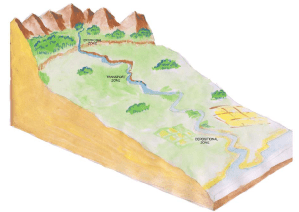FIGURE 1. The three zones of a fluvial system

In the first or upper zone the erosional process predominates and the stream and riverbeds are generally degraded. The streams join together at Confluences and their slopes are generally steep. The bed material is characteristically composed of boulders, cobbles or gravels.

The second (middle) zone is characterized by near equilibrium condition between the inflow and outflow of water and sediment. The bed elevation in this equilibrium zone is fairly constant and the river generally flows in a single channel- there are few confluences or branching (as in the last zone) the sediment material generally composes gravels and sands of various sizes.

The lower zone is characterized by net sedimentation and riverbed aggradations. There is branching of the river into channels and the slope of these channels is rather flat. The bed material generally composes of fine sand to silt and clay.

Sediment erosion, transport and deposition by river

It is amply clear that since rivers play a decisive role in land form evolution, the force of water is intricately connected to the dislodging of soil and rock particles and their conveyance. Where the power of water becomes less, it is forced to deposit the particles on its way.

When water flows over a surface, there exists a shear stress at the interface (Figure 2).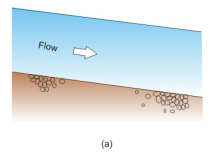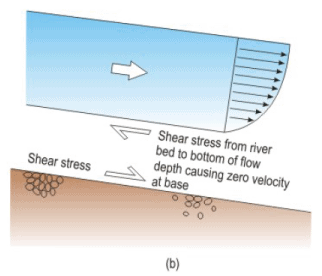Figure 2. Interaction of forces between flow and riverbed (a} General view
(b)Free body diagram showing shear stress at flow base-river bed interface.

Mathematically, the shear stress may be expressed in terms of the flow variables of a flowing river. If S0 is the slope of the channel bed, V is the velocity of flow and h is the water depth at a point then the shear stress (τ0 ) at the interface of the water and the streambed is given as

τ0 = ρghSf                 (1)

Where Sf is the slope of the energy grade line (EGL) shown in the Figure 3. EGL represents the total energy of the stream at any point given by:

E = Z + h + V2/2g                 (2)

For explanation of the terms, one may refer to Lesson 2.8, and hydraulic text books like Ven Te Chow “Open Channel Hydraulics” (1959: McGraw Hill).

If the momentum of the moving water, its hydrostatic pressure its downstream weight component of water and the bottom friction are equated, the following equation results: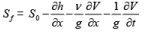(3)

From the equation (3) one may find Sf and evaluate shear stress (τ ) using  equation (1).

In deriving equation (3) it is assumed that the flow variables are not uniform and unsteady if they are not so then the derivatives with respect to the spatial dimension x and time t  vanish, thus simplifying the equation to

Sf = S0              (4)

This is the case of the uniform flow and the corresponding equation for the shear stress (τ0 ) is given as

τ0 = ρ g h S0                  (5)

Thus, knowing the shear stress, it is possible to find out whether it is sufficient to dislodge a particle of diameter ds from the streambed by a method suggested by Shields (Julien, 1995).

First, the dimensionless particle d. is found out by the following formula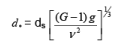(6)

Where G is the specific gravity of the sediment particle;ν is the kinematic viscosity of the fluid and g is the acceleration due to gravity. In some books, G is expressed as Sg.

The ratio of the shear force to bed particle defines the shields parameter τ * as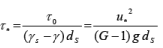(7)

Where τ0 is the boundary shear stress and u* is the shear velocity defined as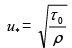(8)

In equation (7), γand γ are the specific weights have the sediment particle and water respectively. Note that τ * depends on τ0 , and which again is the function of S0 (or Sf). Whether τ * is competent enough to dislodge a particle depends on a critical value of shear stress given for particle of given diameter and density as given in Figure 3.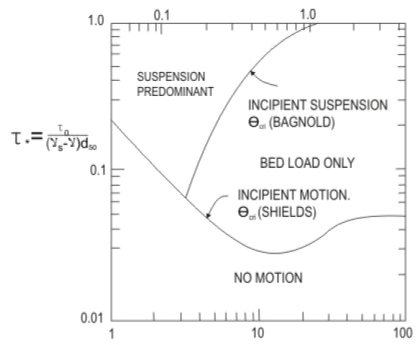Figure 3. Particle motion diagram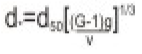d50 : Mean diameter of particle(grain size)
G   : Relative Density of particle
g : Accaleration due to gravidy
ys, : Unit weight of sediment
y : Unit weight of water
v : Kinematic viscosity of water
τ : Shields parameter or entrainment function

The parameter τ *c given in Figure 3 is the critical value of Shields parameter corresponding to the beginning of the motion (τ *c ). This value of shear stress τc of certain particle sizes are as in the following table:

 Particle Type Diameter ds (mm) Critical Shear Stress τc (N/mm 2 ) Cobble 130 111 Grand 8 5.7 Sand 0.25 0.194

Once the sediment particles are dislodged they get carried so long as τ* is larger than τ*c it may be remembered that in a natural stream, uniform flow rarely exists and the flow variables vary along the stream as the cross section and slope changes. However, if the shear stress τ* may be sufficiently large it would continue to convey sediment particles of size smaller than a certain size ds.

Offer running on EduRev: Apply code STAYHOME200 to get INR 200 off on our premium plan EduRev Infinity!

## Water Resources Engineering

158 docs|16 tests

,

,

,

,

,

,

,

,

,

,

,

,

,

,

,

,

,

,

,

,

,

;